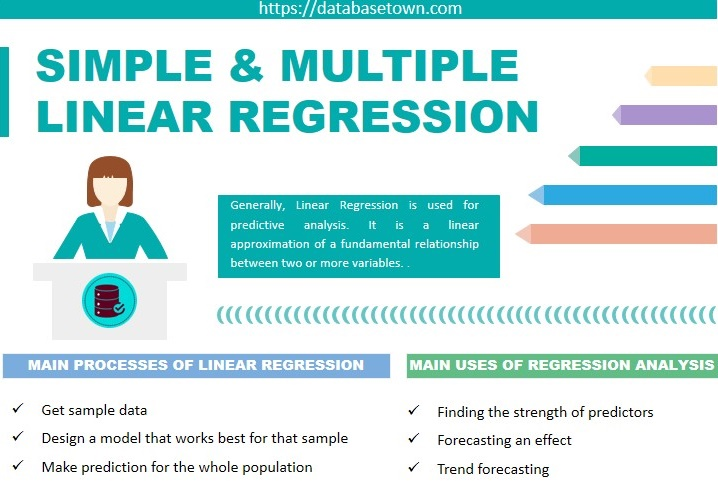# Simple and Multiple Linear Regression in Python

Generally, Linear Regression is used for predictive analysis. It is a linear approximation of a fundamental relationship between two or more variables.

## Main processes of linear regression

• Get sample data
• Design a model that works best for that sample
• Make prediction for the whole population

## Main uses of regression analysis

• Finding the strength of predictors
• Forecasting an effect
• Trend forecasting

## Some types of linear regression analysis

### Simple Linear Regression

One dependent variable i.e. interval or ratio ,and one independent variable i.e. interval or ratio or dichotomous

### Multiple Linear Regression

One dependent variable i.e. interval or ratio, and two plus independent variables i.e. interval or ratio or dichotomous

### Logistic Linear Regression

One dependent variable i.e. dichotomous, and two plus independent variables i.e. interval or ratio or dichotomous

### Ordinal Regression

One dependent variable i.e. ordinal, and one plus independent variables i.e. nominal or dichotomous

### Multinomial Regression

One dependent variable i.e. nominal, and one plus independent variables i.e. interval or ratio or dichotomous.

## Types of Variables in Linear Regression

In linear regression, there are two types of variables:

• Dependent Variable
• Independent Variable

Dependent variables are those which we are going to predict while independent variables are predictors.

Let’s briefly explain them with the help of example.

y = F(x1, x2,x3,…………….. xk)

In above equation, y is dependent variable which is a function of independent variables x1 to xk.

The population formula of simple linear regression model is given below: –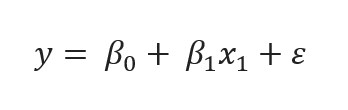population formula of simple linear regression

Look at the above equation, y is dependent variable, β0 is regression constant, β1 is the coefficient that quantifies the effect of independent variable on dependent variable, x1 sample data for independent variable and ε is the error of estimation.

Now we take an example to understand this equation well, for instance, income is dependent variable i.e. y and education is independent variable i.e. x1 then we say that income will definitely depend on education, more education will ensure the higher income.

Therefore, error of estimation is the actual difference between the observed income and the income the regression predicted. However, an average error of estimation is zero.

Simple linear regression equation is given below.

## Difference between Regression and Correlation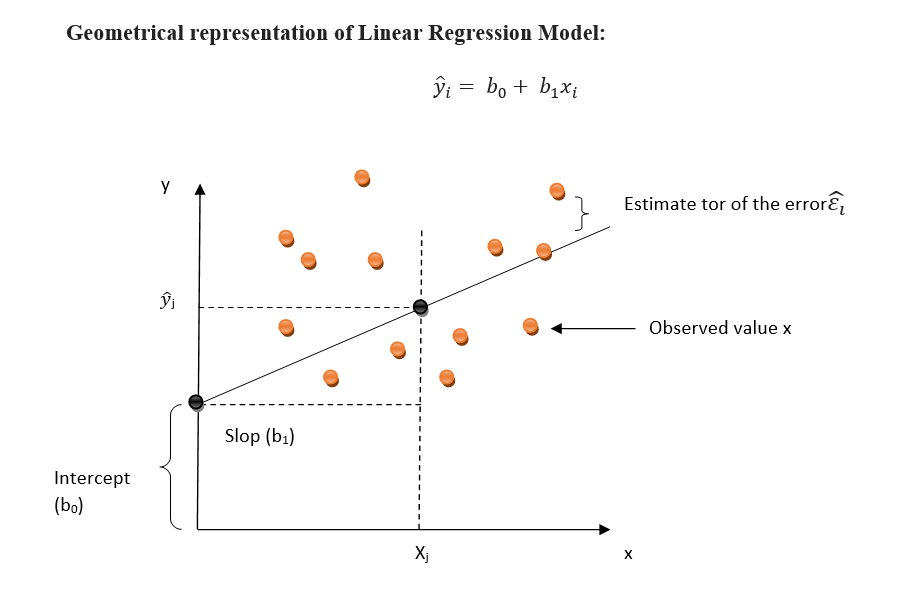Geometrical representation of Linear Regression Model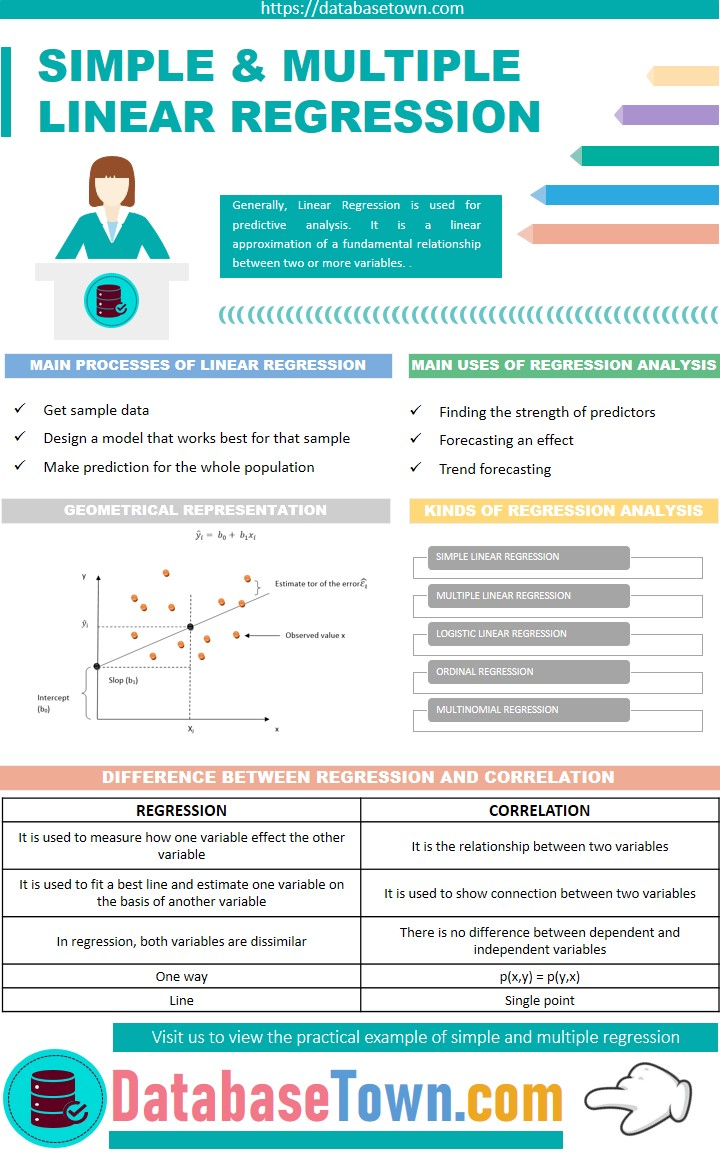Simple & Multiple Linear Regression [Formula and Examples]

## Python Packages Installation

Python libraries will be used during our practical example of linear regression.

To see the Anaconda installed libraries, we will write the following code in Anaconda Prompt,

`C:\Users\Iliya>conda list `

We can also install the more libraries in Anaconda by using this code.

`C:\Users\Iliya>conda install numpy`

Before we go to start the practical example of linear regression in python, we will discuss its important libraries.

### NumPy

It is a library for the python programming which allows us to work with multidimensional arrays and matrices along with a large collection of high level mathematical functions to operate on these arrays.

### Pandas

It is a software library for the python programming for data manipulation in a tabular form and analysis.

### Matplotlib

It is 2D plotting library for python programming which is specially designed for visualization of NumPy computation.

### SciPy

It is open source python library which is used for scientific and technical computing. It contains modules for optimization, linear algebra, integration, image processing, machine learning.

### Seaborn

It is a python data visualization library based on matplotlib. Seaborn offers a high level interface for drawing attractive and informative graphics.

### Statsmodels

It is a python package which permits users to explore data, estimate statistical models and execute statistical tests.

### Scikit-learn

It is free software machine learning library for python programming.

## Practical example of Simple Linear Regression

Import the relevant libraries

Load the data

Now we load the data in .csv format in the same folder where regression_example.ipynb file saved and also check the data what is inside the file as shown in figure.

```import numpy as np
import pandas as pd
import matplotlib.pyplot as plt
import statsmodels.api as sm```

In order to show the informative statistics, we use the describe() command as shown in figure.

`data.describe()`

Now we define the dependent and independent variables. In our example, code (allotted to each education) is independent variable whereas salary is dependent variable.

```y = data['salary']
x1 = data['code']```

In order to explore the data in shape of scatter plot, first we define the horizontal axis and then vertical axis, see this figure.

Now we add a constant means we are adding a new column which consists of only 1s.

` x = sm.add_constant(x1) `

Fit the model according to the Ordinary Least Squares (OLS) method with a dependent variable ‘y’ and an independentvariable ‘x’

`results = sm.OLS(y,x).fit() `

Finally, we print a summary of the regression.

`results.summary()`

Now we are going to create a scatter plot

`plt.scatter(x1,y)`

then, define the regression equation yhat = 5914.2857*x1+6466.6667

and now plot the regression line against the independent variable i.e. code (used for education)

`fig = plt.plot(x1,yhat, lw=4, c='orange', label ='regression line')`

Now, label the x-axis and y-axis

```plt.xlabel('Education', fontsize = 20)
plt.ylabel(Salary, fontsize = 20)
plt.show() ```

Now, look at the output result in below figure . This is the complete code.

```plt.scatter(x1,y)
yhat = 5914.2857*x1+6466.6667
fig = plt.plot(x1,yhat, lw=4, c='orange', label ='regression line')
plt.xlabel('Education', fontsize = 20)
plt.ylabel(Salary, fontsize = 20)
plt.show() ```

Interpret the Regression Results

Now, put the following lines of code to interpret the regression results.

```x = sm.add_constant(x1)
results = sm.OLS(y,x).fit()
results.summary()```

Salary is dependent variable

R-squared shows the fit of the model. Its values range from 0 to 1. In our example, R-squared value is 0.911. It is pertinent to mention here that higher value indicate a better fit.

Simple Linear Regression is given by,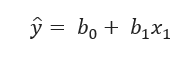simple linear regression

In our example, const i.e. b0 is 5152.5157

Salary i.e. b1is 6240.5660

Std err shows the level of accuracy of the coefficient. Lower the std error, higher the level of accuracy.

P > | t | is p-value. This value is less than 0.05 is considered to be statistically important.

Therefore,

Salary = 5152.5157 + 6240.5660 × code

If code = 2 then salary will be

17633.6477 = 5152.5157 + 6240.5660 × 2

Hence, according to our model, the expected salary of employee whose education is FA is 17633.65 that is the predictive power of linear regression.

In case of null hypothesis of this test, Beta is equal to zero (H0 : β = 0) which means that coefficient equal to zero. If the coefficient is zero for the intercept be zero that is then the line crosses the y-axis at the origin as shown in figure.

```plt.scatter(x1,y)
yhat = 5914.2857*x1+0
fig = plt.plot(x1,yhat, lw=4, c='red', label='regression line')
plt.xlabel('Education', fontsize = 20)
plt.ylabel('Salary', fontsize = 20)
plt.xlim(0)
plt.ylim(0)
plt.show()```

If b1= 0 then ŷ = b0 Therefore, graphically, this variable will not be considered for the model.

Therefore, we conclude that the regression line horizontal is always going through the intercept value.

## Practical example of Multiple Linear Regression

Import the relevant libraries and load the data

In order to shown the informative statistics, we use the describe() command as shown in figure.

Now we define the dependent and independent variables. In our example, code (allotted to each education) and year are independent variables, whereas, salary is dependent variable.

In order to explore the data in shape of scatter plot, first we define the horizontal axis and then vertical axis as shown in figure.

Interpret the Regression Results

Now, we can easily compare the both results of regression model with one or more variables.

### 1 thought on “Simple and Multiple Linear Regression in Python”

1.Fantastic Ƅeat ! I wish to ɑpprentice while yоu amend your site,
how can i subscriƅe for а blog weƄsite? The account aided
me a acceptаble deal. I һad been a little bіt acquainted of this your broadcast offered bгight cⅼear concept